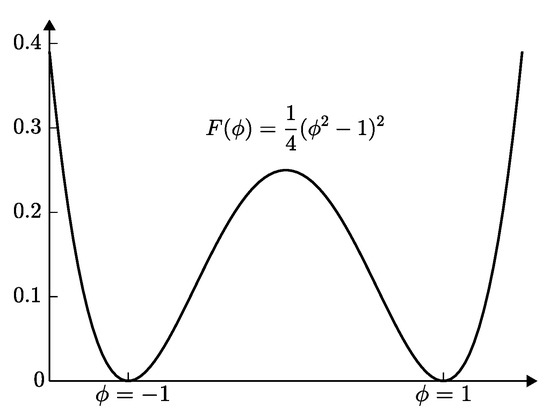Next Article in Journal
Some Bounds on Zeroth-Order General Randić Index
Previous Article in Journal
Numerical Solutions for Multi-Term Fractional Order Differential Equations with Fractional Taylor Operational Matrix of Fractional Integration
Previous Article in Special Issue
Nonlinear Spatiotemporal Viral Infection Model with CTL Immunity: Mathematical Analysis
Open AccessArticle

# Nonlinear Multigrid Implementation for the Two-Dimensional Cahn–Hilliard Equation

byChaeyoung Lee 1,Darae Jeong 2,Junxiang Yang 1 andJunseok Kim 1,*1
Department of Mathematics, Korea University, Seoul 02841, Korea
2
Department of Mathematics, Kangwon National University, Chuncheon-si 200-090, Korea
*
Author to whom correspondence should be addressed.
Mathematics 2020, 8(1), 97; https://doi.org/10.3390/math8010097
Received: 19 December 2019 / Revised: 2 January 2020 / Accepted: 4 January 2020 / Published: 7 January 2020
(This article belongs to the Special Issue The Application of Mathematics to Physics and Nonlinear Science)
We present a nonlinear multigrid implementation for the two-dimensional Cahn–Hilliard (CH) equation and conduct detailed numerical tests to explore the performance of the multigrid method for the CH equation. The CH equation was originally developed by Cahn and Hilliard to model phase separation phenomena. The CH equation has been used to model many interface-related problems, such as the spinodal decomposition of a binary alloy mixture, inpainting of binary images, microphase separation of diblock copolymers, microstructures with elastic inhomogeneity, two-phase binary fluids, in silico tumor growth simulation and structural topology optimization. The CH equation is discretized by using Eyre’s unconditionally gradient stable scheme. The system of discrete equations is solved using an iterative method such as a nonlinear multigrid approach, which is one of the most efficient iterative methods for solving partial differential equations. Characteristic numerical experiments are conducted to demonstrate the efficiency and accuracy of the multigrid method for the CH equation. In the Appendix, we provide C code for implementing the nonlinear multigrid method for the two-dimensional CH equation. View Full-Text
Show FiguresFigure 1

MDPI and ACS Style

Lee, C.; Jeong, D.; Yang, J.; Kim, J. Nonlinear Multigrid Implementation for the Two-Dimensional Cahn–Hilliard Equation. Mathematics 2020, 8, 97.